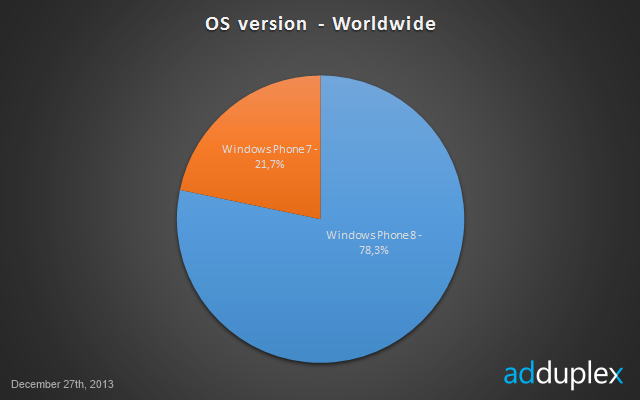You are here
Home > Latest Posts > Opinions > In Q4, 11.8 million WP devices & 10.6 million Lumias may have been sold (On basis of Adduplex stats)!!

# In Q4, 11.8 million WP devices & 10.6 million Lumias may have been sold (On basis of Adduplex stats)!![embedit snippet=”fluids”]

## December stats:## October Stats:Once again on popular demand, we try to predict what Windows Phone volume could have been sold in Q4, 2013. This calculation is based on Adduplex stats. Assuming 30+8= 38 million active Windows Phones till the end of Q3, we see that Windows Phone 8 share has grown further by 7.4% during the period from 11th October to 27th December. So, we will first calculate the volume for this period and then extrapolate it to calculate the volume sold for the entire Q4.

Just to remind you, we predicted 9.7 million Lumia sales for Q3 and actual was 8.8 million!

## Calculation:

Let us assume conservatively that only 0.5 million WP7 devices were sold in Q4. Then considering 40% and 45% skew in Adduplex data in start and end of Q4, we will have = ((38*0.291*1.4)+0.5) / (0.217*1.45) = 50 million devices at the end of Q4.

Hence WP devices sold in Q4=50-40 =10 million devices. Remember, this figure is for some (90-14) = 76 days. Hence the WP devices volume for Q4 should be = 90/76*10 = 11.85 million devices. Nokia may have sold 11.8 *0.9 = 10.6 million Lumia devices.

Disclaimer: This calculation is based on “Adduplex stats” and may vary from actual installed base figures as the apps usage by newer devices is always higher as compared to older devices. Though we use coefficients to correct the skew, but still this calculation is for academic interest only!!